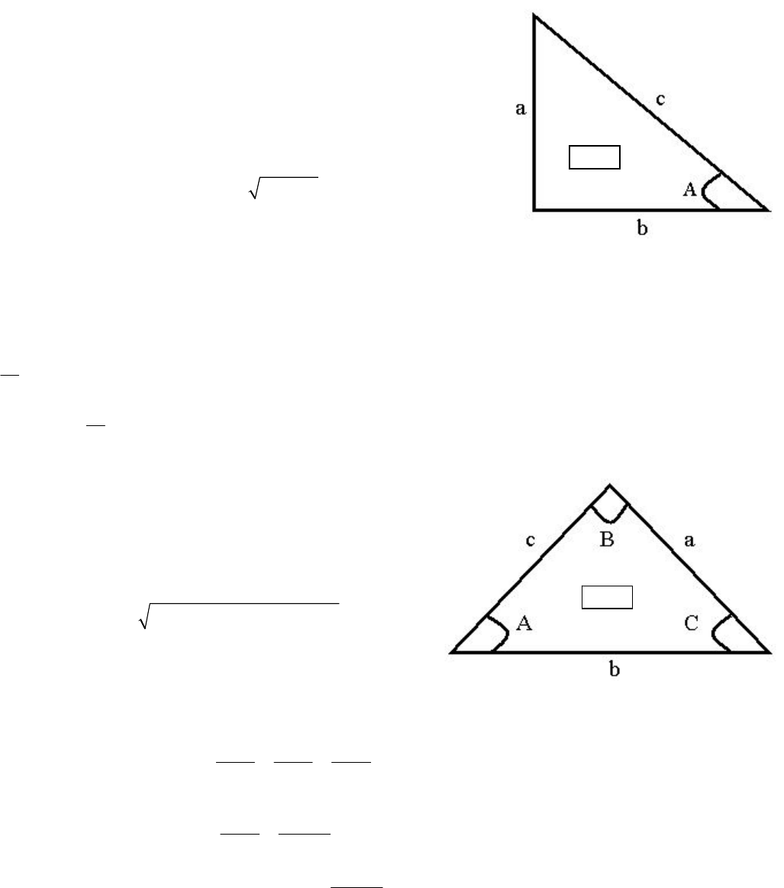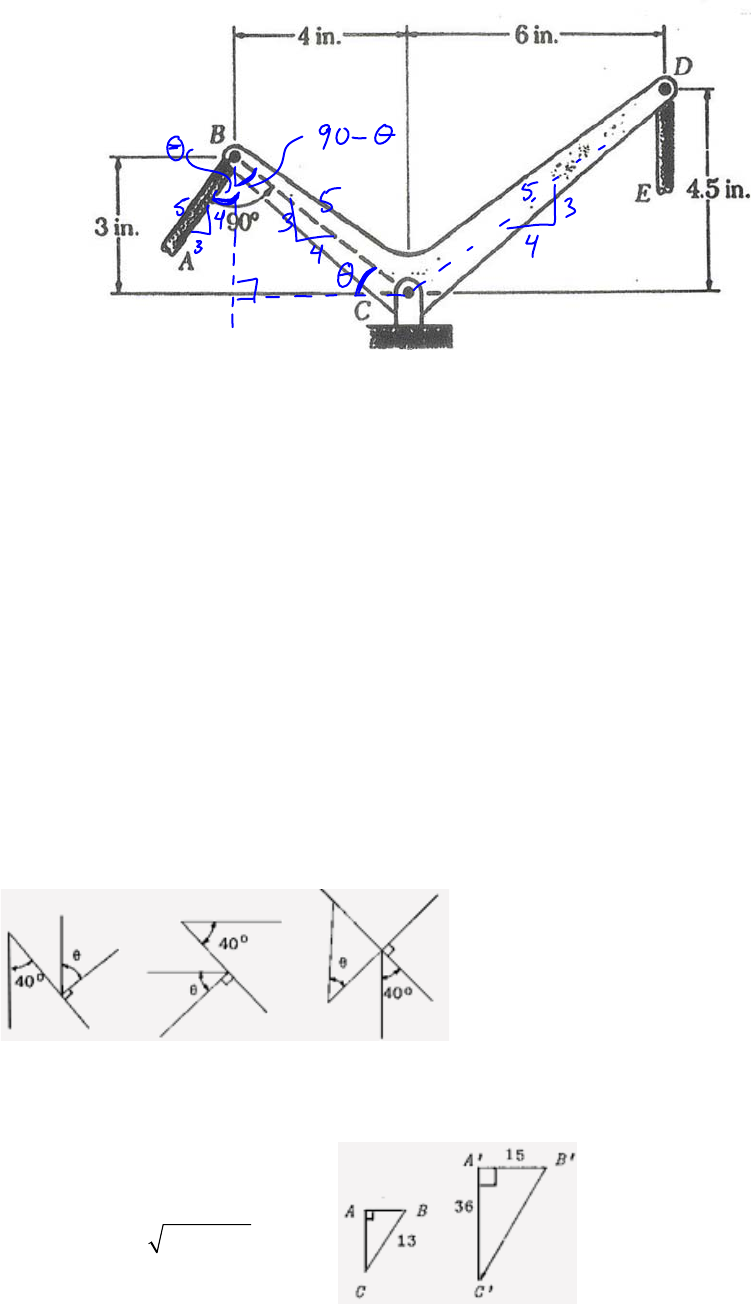# ENGG 202 Lecture Notes - Lecture 1: Hypotenuse, Quadratic Equation, Telephone Numbers In The United Kingdom

49 views6 pagesENGG 202 Assumed Knowledge - Solutions
Q1: For the right angled triangle shown, what is the length of side a?
Side c is 10 m and side b is 6 m.
Solution:
To determine the length of side a,
use Pythagorean’s Theorem (C2 = A2 + B2)
a
2 = 102 – 62
22
10 6 8am=−=
Q2: For the right angle triangle shown, what is angle A in degrees?
Side a is 8 m and side c is 10 m.
Solution:
For a right triangle, the ratio of the opposite side to the hypotenuse is the SIN of the angle.
8sin( )
10
8
arcsin 53.1
10
o
A
A
=
⎛⎞
==
⎜⎟
⎝⎠
Q3: For the non-right triangle shown, what is the length of side a?
Side b is 10 m and side c is 6 m. Angle A is 25 degrees.
Solution: Use the Cosine Law: c2 = a2 + b2 -2abCOS(C)
22
10 6 2(10)(6)cos25 5.22
o
am=+ =
Q4: For the non-right triangle shown, what is the angle C in
degrees? Side a is 5 m and side c is 8 m. Angle A is 30 degrees.
Solution: Use the Sine Law: sin sin sin
abc
A
BC
==
sin sin30
85
sin30
arcsin 8 53.1
5
o
oo
C
mm
Cm
=
⎛⎞
==
⎜⎟
⎝⎠
Q1,Q2
Q3,Q4
Unlock document

This preview shows pages 1-2 of the document.
Unlock all 6 pages and 3 million more documents.Q5: For the lever shown, and without calculating any angles:
a) Determine the lengths of arms BC and CD.
b) Find the coordinates of the midpoint of arm BC?
(assume C is 0,0)
c) If link AB is 2 in long, find the coordinates of
point A.
Solution:
a) either from Pythagorean’s rule or recognizing that both BC and CD are the hypotenuse of a 3-
4-5 triangle, the length BC is 5 in, and CD is 7.5 in (CD is a 3-4-5 triangle with multiples of
1.5 in).
b) The midpoint of BC is located halfway between B and C. since C is the 0,0 point and B is
located at (-4, 3) in, then the midpoint is located at (-2, 1.5) in.
c) Since BC is a 3-4-5 triangle with the 3 on the vertical and the 4 on the horizontal, the line
perpendicular to it (AB) is also a 3-4-5 with the 3 and 4 reversed (3 on the horizontal, 4 on the
vertical). In this case the hypotenuse has length 2 in and the ratios are: 2in/5 = x/3 = y/4
therefore the horizontal dimension of AB (x) is 1.2 in and the vertical dimension is 1.6 in.
The coordinates of point A are therefore (-4-1.2, 3-1.6) = (-5.2, 1.4) in.
If using the ratios is too confusing, then ensure you can solve it using the angles. Using the ratios
can just save you a bit of time if you know how to use them.
Q6: Determine the angle θ
You have to assume parallel lines or it is impossible to solve.
In all cases, the angle is 50o.
Q7: Determine the length of side AB if right angle ABC is
similar to right angle A'B'C'.
First determine the hypotenuse of A’B’C’: 22
15 36 39
=
+=
Using ratios: AB/13 = 15/39 AB = 5
AC/13 = 36/39 AC = 12
Unlock document

This preview shows pages 1-2 of the document.
Unlock all 6 pages and 3 million more documents.